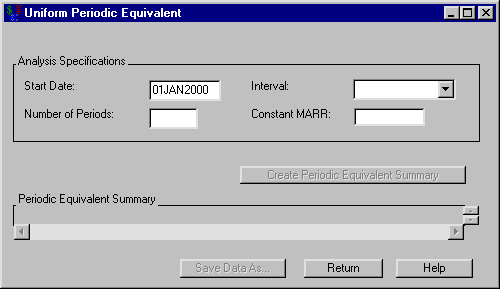# Analyses

### Uniform Periodic Equivalent

Having selected a generic cashflow from the Investment Analysis dialog box, to perform a uniform periodic equivalent, select AnalyzePeriodic Equivalent from the Investment Analysis dialog box’s menu bar. This opens the Uniform Periodic Equivalent dialog box displayed in Figure 58.10.

Figure 58.10: Uniform Periodic Equivalent Dialog BoxThe following items are displayed:

Analysis Specifications

Start Date holds the date the uniform periodic equivalents begin.

Number of Periods holds the number of uniform periodic equivalents.

Interval holds how often the uniform periodic equivalents occur.

Constant MARR holds the Minimum Attractive Rate of Return.

Create Periodic Equivalent Summary becomes available when you adequately fill the Analysis Specification area. Clicking Create Periodic Equivalent Summary then fills the periodic equivalent summary.

Periodic Equivalent Summary fills with two columns when you click Create Periodic Equivalent Summary. The first column lists the investments selected. The second column lists the computed periodic equivalent amount.

Print becomes available when you fill the periodic equivalent summary. Clicking it sends the contents of the summary to the SAS session print device.

Save Data As becomes available when you generate the periodic equivalent summary. Clicking it opens the Save Output Dataset dialog box where you can save the summary (or portions thereof) as a SAS Dataset.

Return takes you back to the Investment Analysis dialog box.